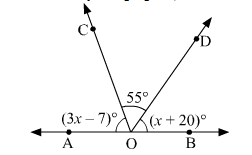# In the adjoining figure, AOB is a straight line.

Question:

In the adjoining figure, AOB is a straight line. Find the value of x. Hence, find ∠AOC and ∠BOD.Solution:

As $A O B$ is a straight line, the sum of angles on the same side of $A O B$, at a point $O$ on it, is $180^{\circ}$.

Therefore,

$\angle \mathrm{AOC}+\angle \mathrm{COD}+\angle \mathrm{BOD}=180^{\circ}$

$\Rightarrow(3 x-7)^{\circ}+55^{\circ}+(x+20)^{\circ}=180$

$\Rightarrow 4 x=112^{\circ}$

$\Rightarrow x=28^{\circ}$

Hence,

$\angle \mathrm{AOC}=3 x-7$

$=3 \times 28-7$

$=77^{\circ}$

and $\angle \mathrm{BOD}=x+20$

$=28+20$

$=48^{\circ}$